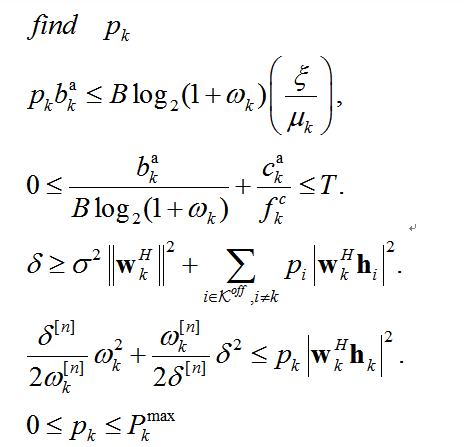# How do you solve this problem? Status: Infeasible Optimal value (cvx_optval): +Inf

Hello everyone, I have encountered this problem while writing the code, I have tested my code, some places are orders of magnitude (E10 / E-11), I also checked the forum posts and tried to normalize the operation and replace the solver, but still have the above problem, please help meThat’s the problem I’m trying to solveThis is my input

N = 4;
M = 20;
K = 5;
B = 10e6;
mu = 1;
savedata = 0;
%---------powers------------
noise_maxpower_original = 10^((-80-30) / 10);
% noise_maxpower = 1;
trans_maxpower =2 ;
%—computing limitation------
F_local_max= 1e9;
F_c_max = 20e9;
k_energy = 1e-28;
T_MAX = 3;
for ii = 1 : K
user_data_amount(ii) =randi([250 350])* 1024 * 8;
user_circle(ii) = 500* randi([250 350])* 1024 * 8;
end
%% Simulation loop %%%%%%%%%%%%%%%%%%%%%%%%%%%%%%%%%%%%%%%%%%%%%%
%%—input channels------
num_loop = 1;
for loop = 1 : num_loop
outerflag=1;
T1=cputime; %
tic;
H_UB=H_UB_all(1:N,1:K,loop) /sqrt(noise_maxpower_original);%^2：e17
H_UI =H_UI_all(1:M,1:K,loop) /sqrt(noise_maxpower_original);
H_IB = H_IB_all(1:N,1:M,loop) /sqrt(noise_maxpower_original);
noise_maxpower=1;
%%%%%%%%%%%%%%%%%%%%%%%%%%%%%%%%%%%%%%%%%
%%%%% Initialize
A = zeros(K, 1);
phi_temp=randn(M,1) + sqrt(-1)* randn(M,1);
phi_ini=exp(1jangle(phi_temp));
W_ini=randn(N,K);
W(:, :, 1)=full(W_ini);
phi(:, 1)=phi_ini;
Phi(:, :, 1) = diag((phi(:, 1))’);
Phi_ini = Phi(:, :, 1);
trans_power_ini=rand(K,1 );
trans_power (:, 1)=trans_power_ini;
F_c_ini = rand(K, 1)
21e9;
F_c(:, 1) = F_c_ini;
a_local = [];
for ii = 1 : K
if user_circle(ii)<= T_MAX * F_local_max
a_local = [a_local; ii];
end
A(a_local) = 1;
end
E_local = [];
for ii = 1 : length(a_local)
E_local(a_local(ii)) = k_energy
(user_circle(ii)).^3/T_MAX^2; %本地计算能耗
end
E_local;
num_iterative = 300;
for n = 1 : num_iterative
xi_mintrans_power = 0;
xi_maxtrans_power =max(E_local);
%%%%% Optimize P and xi %%%%%
while 1
a_off = [];
xi_trans_power = (xi_maxtrans_power + xi_mintrans_power )/2;
for ii = 1 : length(a_local)
if E_local(a_local(ii)) > xi_trans_power
a_off = [a_off;a_local(ii)];
end
end
a_off ;
for ii = 1:length(a_off)
d(a_off(ii)) = user_circle(a_off(ii))/ F_c_ini(a_off(ii));
end
relax_scaler_delta = 1;
relax_scaler_omega = zeros(K,1);
relax_scaler_omega(a_off) = 1;
[trans_power_1, innerflag] = Generate_trans_power(B, K, a_off (:,n ),H_UI, H_IB, H_UB,…
noise_maxpower_original, trans_maxpower, Phi(:, :, n), W(:, :, n), F_c(:, n ), xi_trans_power, user_data_amount, user_circle, T_MAX, mu, relax_scaler_delta , relax_scaler_omega );

This is the function I made a mistake with

function [trans_power_opt, flag_P] = Generate_trans_power(B, K, a_off, H_UI, H_IB, H_UB,…
noise_maxpower_original,trans_maxpower ,Phi_ini, W_ini , F_c_ini, xi_trans_power, user_data_amount, user_circle, T_MAX , mu, relax_scaler_delta , relax_scaler_omega)
relax_scaler_delta_ini = relax_scaler_delta;
relax_scaler_omega_ini = relax_scaler_omega;
cvx_begin
variable trans_power_opt(K)
variable relax_scaler_omega(K)
variable relax_scaler_delta
for ii = 1:length(a_off)
a1(a_off(ii)) = (norm((W_ini(:, a_off(ii))’( H_IB Phi_iniH_UI(:, a_off(ii)) + H_UB(:, a_off(ii)))), 2)^2);%e10
y(a_off(ii))=((trans_power_opt(a_off(ii))norm((W_ini(:, a_off(ii))’( H_IB
Phi_iniH_UI(:, a_off(ii)) + H_UB(:, a_off(ii)))), 2)^2))(noise_maxpower_original);
end
for ii = 1:length(a_off)
a2(a_off(ii)) = norm(W_ini(:, a_off(ii))’, 2)^2;
z_ini(a_off(ii))=((sum(y) - y(a_off(ii)))+((noise_maxpower_original)^2)norm(W_ini(:, a_off(ii))’, 2)^2) ;
end
for ii = 1:length(a_off)
constraint_2a(a_off(ii)) = trans_power_opt(a_off(ii))user_data_amount(a_off(ii)) - (B(log(1+relax_scaler_omega(a_off(ii))))/log(2))
(xi_trans_power/mu);
constraint_2b(a_off(ii)) = user_data_amount(a_off(ii))inv_pos(Blog(1+ relax_scaler_omega(a_off(ii)) )/log(2)) + user_circle(a_off(ii))/ F_c_ini(a_off(ii)) - T_MAX;
constraint_3(a_off(ii)) = relax_scaler_delta - z_ini(a_off(ii));
constraint_4(a_off(ii)) = (relax_scaler_delta_ini/(2*relax_scaler_omega_ini(a_off(ii)))) relax_scaler_omega(a_off(ii))^2+ (relax_scaler_omega_ini(a_off(ii))/ (2relax_scaler_delta_ini))*relax_scaler_delta^2 - y(a_off(ii));
end
subject to
for ii = 1:length(a_off)
real(constraint_2a) <= 0;
real(constraint_2b)<= 0 ;
real(constraint_3) >= 0;
real(constraint_4)<= 0;
0 <= trans_power_opt <= trans_maxpower;
relax_scaler_omega >= 0;
relax_scaler_delta >= 0;
end
cvx_end

1 Like

In addition to improving numerical scaling, follow the advice in https://yalmip.github.io/debugginginfeasible , except for section 1,which does not apply to CVX.

Is this just a feasibility problem? I guess so, If so, then section 2 of the link does not apply. So follow the advice in sections 3 and later.

Ok,Thanks,but before that, I think I may not understand the idea of normalization, could you give me an example to illustrate it

I always thought it might be an order of magnitude problem, and when I took some very large numbers and made them the same order of magnitude as other numbers, the problem worked, but the results didn’t seem accurate

For example, instead of 10^8 mm, use 10^2 km. You have to make appropriate changes throughout you r model so that it is correct with the new units.

OK, thank you very much